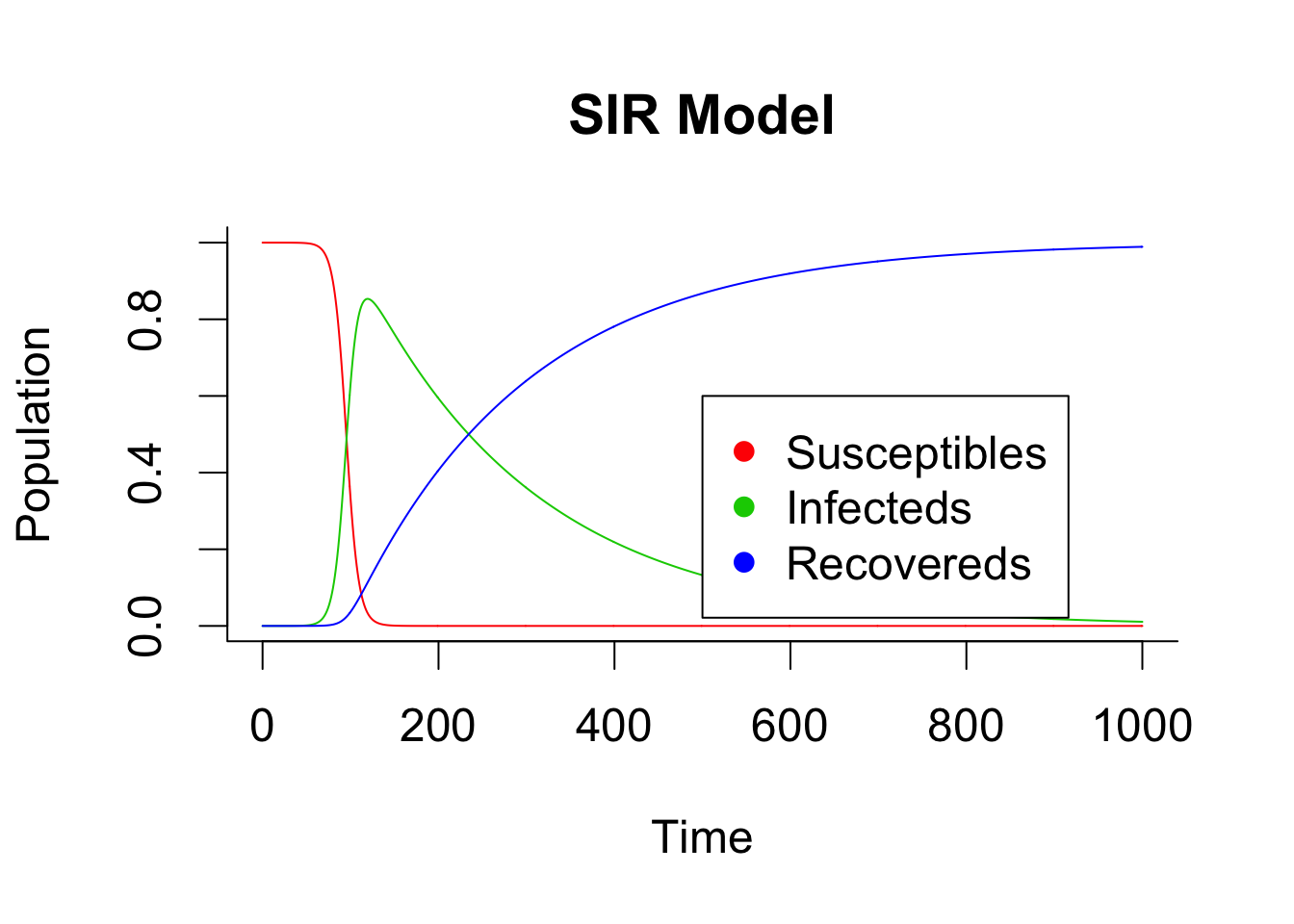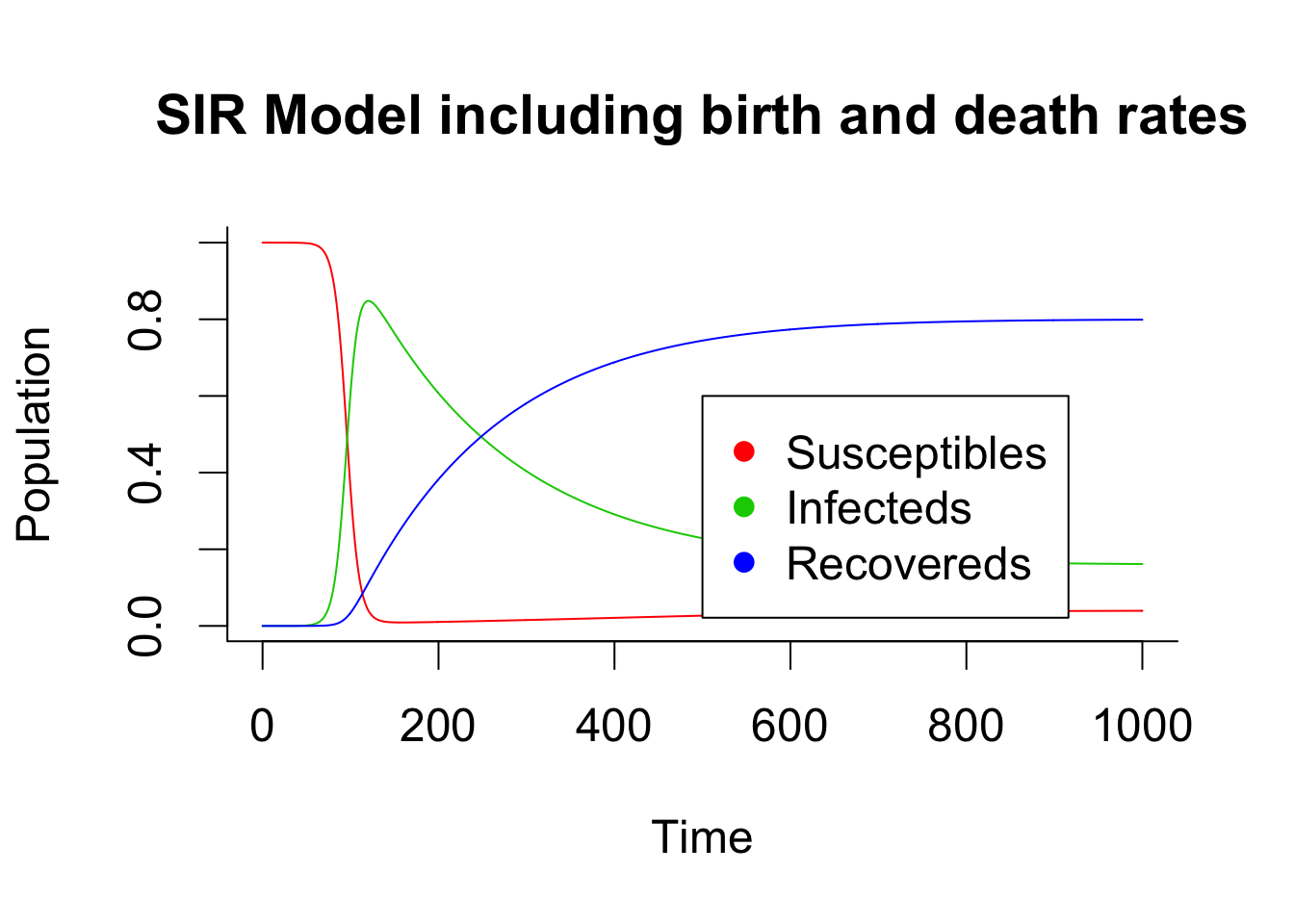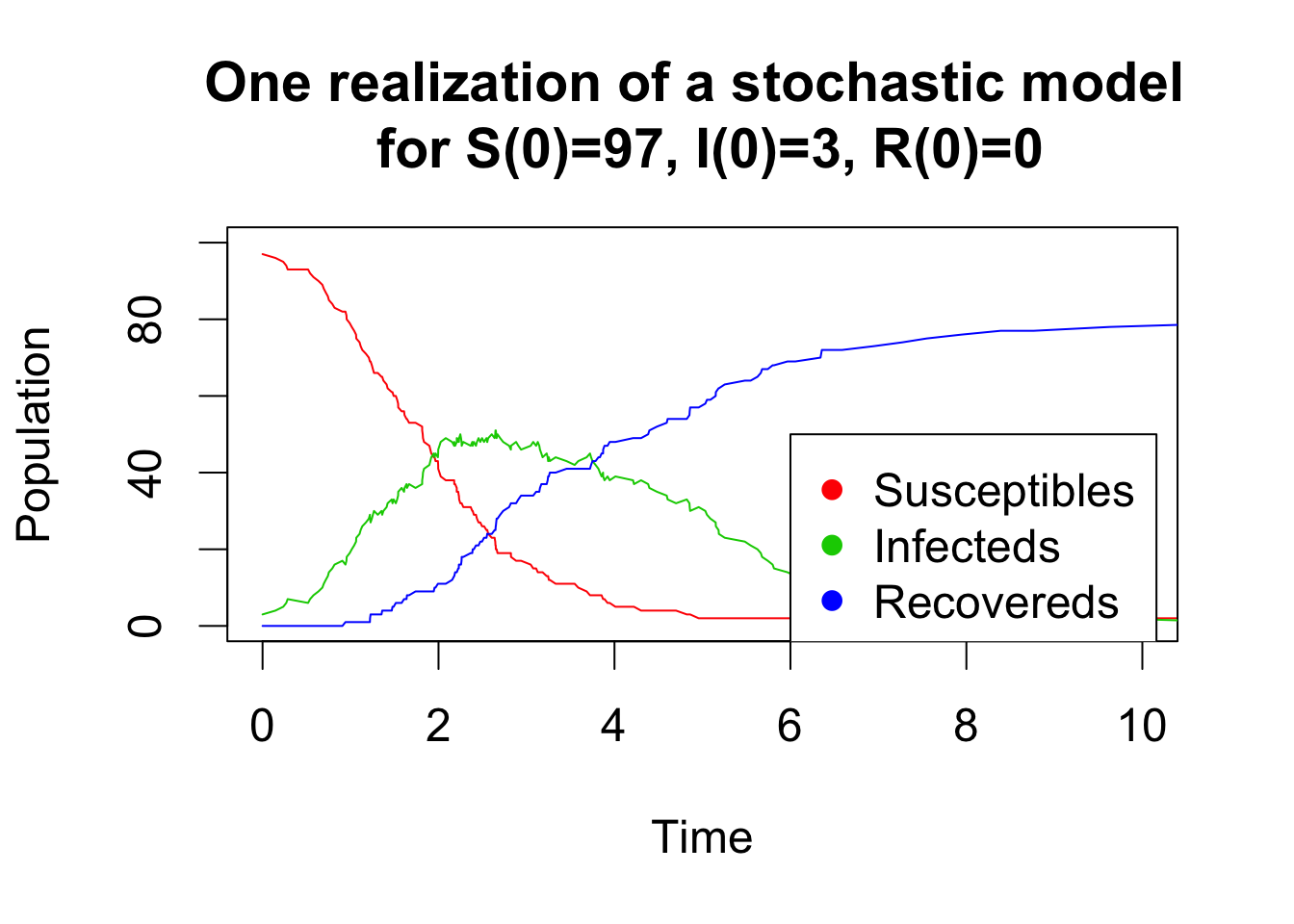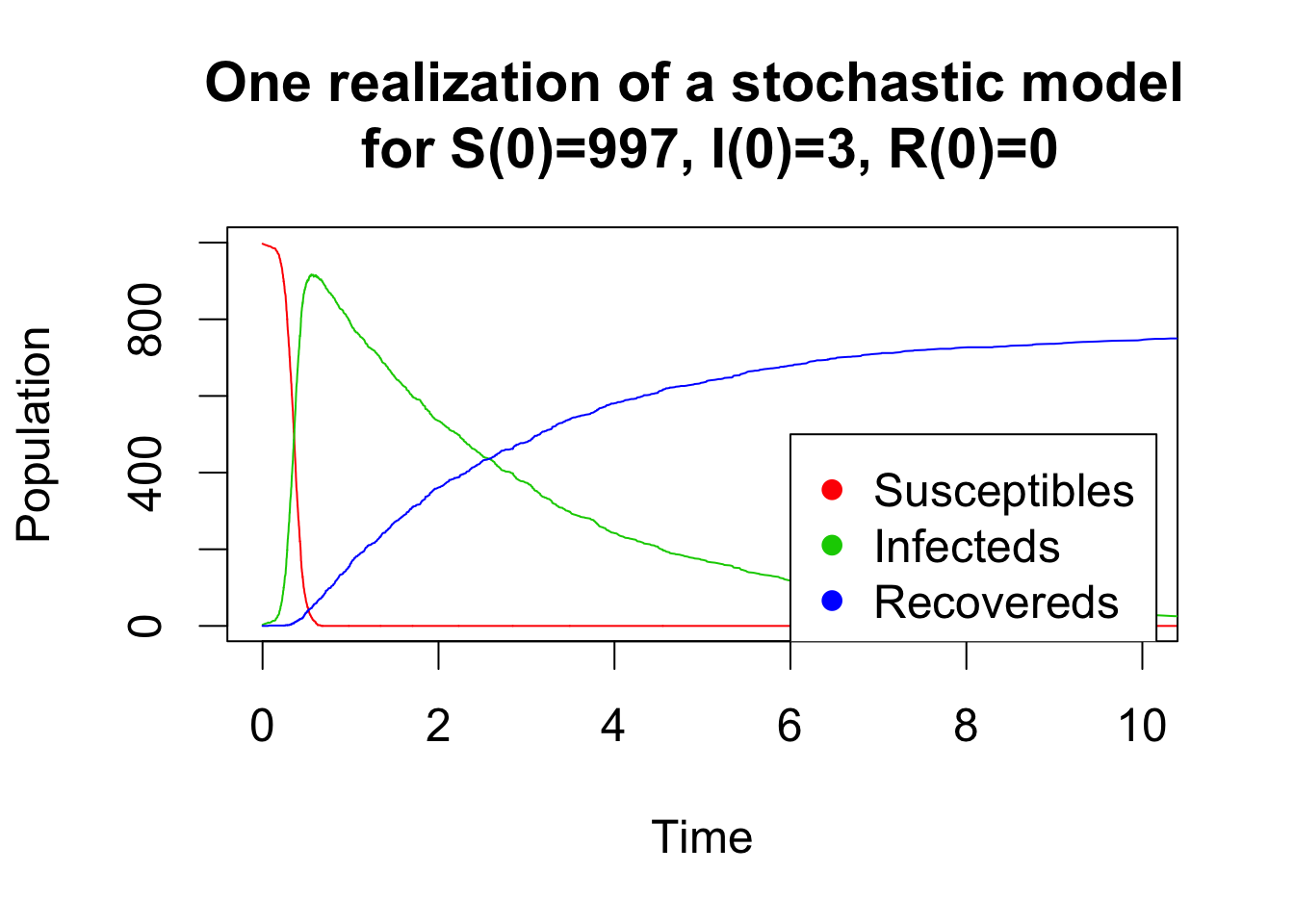# Stochastic SIR

I did my Master Thesis, about Infectious Disease Inference, at Stockholm University in the departement of Mathematical Statistics. Infectious disease inference is a very interesting and challenging research subject. Moreover this domain of statistcs is at the edge between statistics and simulations (which made my background in physics very relevant and suddenly make my stats and physics master start resonating). I think that this was my very first contact with computational statistics and it gave me the desir to learn more!

Here is a little bit of epidemic modelling. A detailed version (with references, codes and examples) of this introduction can be found here.

# SIR model

The mathematical modelling of infectious diseases (aka Infectious Disease Inference) is a tool to study the mechanisms by which diseases spread, to predict the future course of an outbreak and to evaluate strategies to control an epidemic. In the class of compartmental models, which serve as a base mathematical framework for understanding the complex dynamics of infectious diseases, the Susceptible-Infectious-Recovered (SIR) model is a valuable model for many infectious diseases.

## Deterministic setting

When dealing with large populations deterministic models are often used. In deterministic compartmental models, the transition rates from one compartment to another are mathematically expressed as derivatives. Hence the model is formulated using ordinary differential equations (ODE). A closed population is assumed at all times $$t$$, then $$P(t)=S(t)+I(t)+R(t)$$.

• $$\frac{dS(t)}{dt} = - \beta S(t)\frac{I(t)}{P(t)}$$
• $$\frac{dI(t)}{dt} = \beta S(t)\frac{I(t)}{P(t)} - \gamma I(t)$$
• $$\frac{dR(t)}{dt} = \gamma I(t)$$

Here are two example of deterministic modelling with and without birth and death process in the compartments.

##package
library(deSolve)

##################################################
### Basic model SIR simulations
###################################################

##function
sir <- function(time, state, parameters) {
with(as.list(c(state, parameters)), {
dS <- -beta * S * I
dI <- beta * S * I - gamma * I
dR <- gamma * I

return(list(c(dS, dI, dR)))
})
}

##############################################################################
init <- c(S = 1-1e-6, I = 1e-6, 0.0)
parameters <- c(beta = 0.15, gamma = 0.005)
times <- seq(0, 1000, by = 1)
out <- as.data.frame(ode(y = init, times = times, func = sir, parms = parameters))
out$time <- NULL par(cex=1.5) matplot(times, out, type = "l", xlab = "Time", ylab = "Population", main = "SIR Model", lwd = 1, lty = 1, bty = "l", col = 2:4) legend(x = 500,y = 0.6,c("Susceptibles", "Infecteds", "Recovereds"), pch = 16, col = 2:4)################################################## ### Model SIR with birth and dead rate ################################################### sir_bd <- function(time, state, parameters) { with(as.list(c(state, parameters)), { dS <- birth - beta*S*I - death*S dI <- beta*S*I - gamma*I - death*I dR <- gamma*I - death*R return(list(c(dS, dI, dR))) }) } ############################################################################## init <- c(S = 1-1e-6, I = 1e-6, R = 0.0) parameters <- c(beta = 0.15, gamma = 0.005, birth =0.001, death=0.001) times <- seq(0, 1000, by = 1) out <- as.data.frame(ode(y = init, times = times, func = sir_bd, parms = parameters)) out$time <- NULL

matplot(times, out, type = "l", xlab = "Time", ylab = "Population", main = "SIR Model including birth and death rates", lwd = 1, lty = 1, bty = "l", col = 2:4)
legend(x = 500,y = 0.6,c("Susceptibles", "Infecteds", "Recovereds"), pch = 16, col = 2:4)## Stochastic modelling

A stochastic SIR model is defined analogously as the deterministic model. A closed homogeneous population is assumed, and $$S(t)$$, $$I(t)$$ and $$R(t)$$ have the same definition as in the deterministic setting. As done previously, birth and death are ignored in this simple setting. The dynamic of the model is defined as follows. Infectives have contact with susceptibles at a constant rate $$\beta$$. Contacts are mutually independent. Any susceptible which is in contact with an infected individual immediately becomes infective and starts spreading the disease following the same rules. Infected individuals remain infectious for a random amount of time governed by an infections period distribution after which they stop being infectious and recover. The infectious periods are defined to be independent and identically distributed (also independent of the contact processes). The exponential distribution with intensity parameter $$\gamma$$ has received special attention in the literature, because the resulting model is Markovian. A stochastic process is said to be a Markov process or have the markov property if the conditional probability of the future state conditional on both present and past states depends only on the present state of the process.

A simple and efficient algorithm to simulate a stochastic model is the Gillespie algorithm. This algorithm assumes that all possible transitions between compartments occur independently and are simulated at each time step with constant probability per unit time that depends on the current state of the system (i.e the number of individual in each compartment). The idea is to sample the next time event from an exponential distribution. Then, the event is randomly chosen amongst the possible transitions between compartments with probabilities proportional to their individual rates. The code below shows two realizations for a small and a large population of a stochastic SIR model simulated by a Gillespie algorithm.

parms=c(m=0,b=0.02,v=0.1,r=0.3)

# define how state variables S, I and R change for each process
processes <- matrix(c(1,1,1,
-1,0,0,
0,-1,0,
0,0,-1,
-1,1,0,
0,-1,0,
0,-1,1), nrow=7, ncol=3, byrow = TRUE,
dimnames=list(c("birth",
"death.S",
"death.I",
"death.R",
"infection",
"death.infec",
"recovery"),
c("dS","dI","dR")))

##import necessary files/fct
source(file="RFun/gillespie_fct_R.R")

##small population
initial.state=c(S=97, I=3, R=0)

res<-gillespie(parms=parms, X0=initial.state, time.window=c(0,1000), processes=processes,pb = FALSE)
par(cex=1.5)
matplot(x = res[,1], y = res[,-1], type = "l", lty = 1, xlab = "Time", ylab = "Population",col = 2:4, ylim=c(0,100), xlim=c(0,10), main="One realization of a stochastic model \n for S(0)=97, I(0)=3, R(0)=0")
legend(x=6,y=50,c("Susceptibles", "Infecteds", "Recovereds"), pch = 16, col = 2:4)##huge population
initial.state=c(S=997, I=3, R=0)

res<-gillespie(parms=parms, X0=initial.state, time.window=c(0,10000), processes=processes,pb = FALSE)
matplot(x = res[,1], y = res[,-1], type = "l", lty = 1, xlab = "Time", ylab = "Population",col = 2:4, ylim=c(0,1000), xlim=c(0,10), main="One realization of a stochastic model \n for S(0)=997, I(0)=3, R(0)=0")
legend(x=6,y=500,c("Susceptibles", "Infecteds", "Recovereds"), pch = 16, col = 2:4)In a next post I will comment on the code of the parallel gillespie R function …